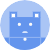search
Search
menu search toc more_vert
Guest 0reps
Thanks for the thanks!
close
account_circle
Profile
exit_to_app
Sign out
help Ask a question
search
keyboard_voice
close
Searching Tips
Search for a recipe:
"Creating a table in MySQL"
Search for an API documentation: "@append"
Search for code: "!dataframe"
Apply a tag filter: "#python"
Useful Shortcuts
/ to open search panel
Esc to close search panel
to navigate between search results
d to clear all current filters
Enter to expand content previewDoc SearchCode Search BetaSORRY NOTHING FOUND!
mic
Start speaking...Voice search is only supported in Safari and Chrome.
Shrink
Navigate to
A
A
brightness_medium
share
arrow_backShareTwitterFacebook

# Appending rows to a Pandas DataFrame

Pandas
chevron_right
Cookbooks
chevron_right
DataFrame Cookbooks
chevron_right
Row and Column Operations Cookbook
schedule Jul 1, 2022
Last updated
local_offer PythonPandas
Tags
expand_more

To append a row (a `list` or `Series`) to a Pandas DataFrame, use the DataFrame's `append(~)` method.

WARNING

For performance, instead of appending rows one by one, opt to append rows at once.

# Appending a single row

Consider the following DataFrame:

``` df = pd.DataFrame({"A":[3,4],"B":[5,6]})df    A  B0  3  51  4  6 ```

## From a list

To use `append(~)`, we first need to convert our row, which is initially represented as a `list`, to a `Series`:

``` my_row = [10,11]s = pd.Series(my_row, index=df.columns)df = df.append(s, ignore_index=True)df A B0 3 51 4 62 10 11 ```

Here, note the following:

• the index of the Series `s` has been set to the column labels of `df` (`A` and `B`) - otherwise, the `append(~)` method won't vertically stack them.

• we set `ignore_index=True` to ignore the index of `s` - otherwise, we would have duplicate values in the new index `0 1 0`.

• a new DataFrame is returned, and the original `df` is kept is intact.

## From a Series

Consider the same DataFrame:

``` df = pd.DataFrame({"A":[3,4],"B":[5,6]})df A B0 3 51 4 6 ```

Suppose we wanted to append the following Series to `df`:

``` s = pd.Series([10,11], index=df.columns)s A 10B 11dtype: int64 ```

Here, notice how the index of the Series has been set as the column labels - otherwise, the `append(~)` method won't vertically stack them.

Now, call `append(~)` like so:

``` df.append(s, ignore_index=True) A B0 3 51 4 62 10 11 ```

The `ignore_index=True` is required because we did not assign a `name` to our Series `s`, and so Pandas does not know what row label to use when it is added to the DataFrame. Passing `ignore_index` tells Pandas to use the default integer index, which in this case is `2`.

# Appending multiple rows

Consider the same `df` as above:

``` df = pd.DataFrame({"A":[3,4],"B":[5,6]})df A B0 3 51 4 6 ```

## From a nested list

To append multiple rows that are represented as a nested list:

``` my_list = [[10,11],[12,13]]df.append(pd.DataFrame(my_list, columns=df.columns), ignore_index=True) A B0 3 51 4 62 10 113 12 13 ```

Here, we are first creating an intermediate DataFrame out of `my_list`:

``` pd.DataFrame(my_list, columns=df.columns) A B0 10 111 12 13 ```

We then call `append(~)` to vertically stack the DataFrames. The `ignore_index=True` tells Pandas to use the default integer index in the resulting DataFrame - otherwise we would have gotten duplicate index `[0,1,0,1]`.

## From a list of Series

To append multiple rows represented as a list of Series:

``` my_series = [pd.Series([10,11], index=df.columns), pd.Series([12,13], index=df.columns)]df.append(my_series, ignore_index=True) A B0 3 51 4 62 10 113 12 13 ```

Notice how, in this case, we do not need to create an intermediate DataFrame. This is because `append(~)` can handle a list of Series by default.

mail